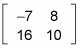##### Linear Algebra For Dummies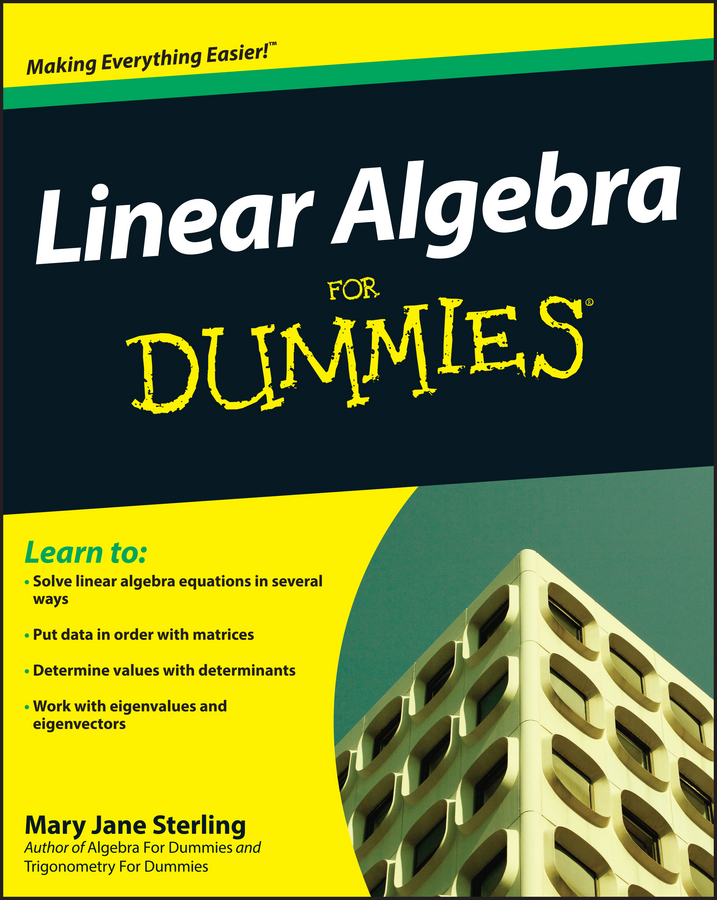When you apply basic operations to matrices, it works a lot like operating on multiple terms within parentheses; you just have more terms in the "parentheses" to work with. Just like with operations on numbers, a certain order is involved with operating on matrices. Multiplication comes before addition and/or subtraction. When multiplying by a scalar, a constant that multiplies a quantity (which changes its size, or scale), each and every element of the matrix gets multiplied.

When adding or subtracting matrices, you just add or subtract their corresponding terms. It's as simple as that. This figure shows how to add and subtract two matrices.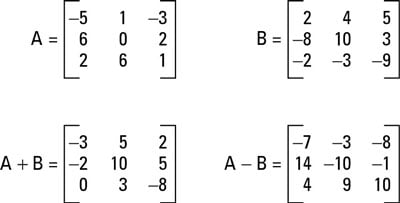Note, however, that you can add or subtract matrices only if their dimensions are exactly the same. To add or subtract matrices, you add or subtract their corresponding terms; if the dimensions aren't exactly the same, then the terms won't line up. And obviously, you can't add or subtract terms that aren't there!

When you multiply a matrix by a scalar, you're just multiplying by a constant. To do that, you multiply each term inside the matrix by the constant on the outside. Using the same matrix A from the previous example, you can find 3A by multiplying each term of matrix A by 3. This example is shown here: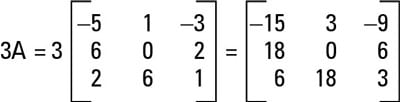Multiplying matrix A by 3.

Suppose a problem asks you to combine operations. You simply multiply each matrix by the scalar separately and then add or subtract them. For example, consider the following two matrices: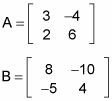Find 3A – 2B as follows:

1. Insert the matrices into the problem.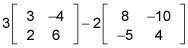2. Multiply the scalars into the matrices.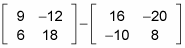3. Complete the problem by adding or subtracting the matrices.